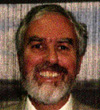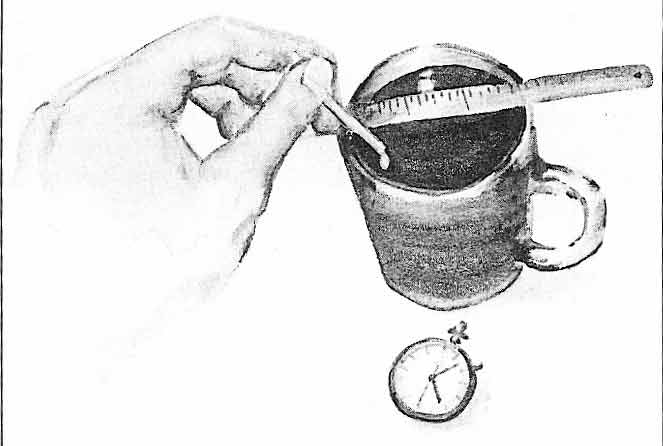## Experiment objective

To simulate how meteorologists track storm clouds.

## You need

• A cup of black coffee (preferably a clear glass cup).
• A little bit of milk or cream.
• A ruler.
• A watch or stopwatch.

In this experiment you will track a drop of white milk as it moves in a cup of very gently stirred black coffee. Imagine that the coffee represents the motion of air in the atmosphere. The drop of milk in the coffee represents a trace gas. By following the motion of the milk, knowing where you dropped it, and using a stopwatch to measure the passage of time, you can determine the velocity or speed of the moving coffee.It’s important to stir the coffee very, very slowly. Before you drop in the milk from the end of a stir stick, lay a ruler across the top of the cup and measure the exact distance in centimetres from the centre of the cup to the spot where the drop will fall. This is the radius (r). Before you let the drop of milk fall, get ready with the stopwatch so you can time exactly how long it takes to go all the way around the cup and back to where it started. When the experiment is over you should have two numbers: r, the radius of the circle, and t, the time it takes to go around the circle one time. You can use the formula 2(pi)r to get the distance the milk travels in one trip around the cup—one rotation. (Pi is a mathematical constant that is roughly 3.14159, so 2(pi) is about 6.28. If the radius you measure is 2cm, then the distance the drop travels in one rotation would be 6.28 x 2 = 12.56cm.) The speed of the slowly moving mixture is the distance divided by the time, so divide the distance the milk travels (say 12.56 cm) by the time, (say 5 seconds) to get 2.51 cm/sec. Now you try your own measurements and calculations. How good are your velocity measurements when the milk and coffee become well mixed?

This is how meteorologists work out the speed of moving clouds, winds and storms in the upper atmosphere. They have satellites that can “see” certain naturally occurring gasses in the atmosphere. One such gas is natural gas, or methane, that sometimes seeps from bogs. Meteorologists use the satellites to “track” this methane as it moves about in the atmosphere, just like you tracked the milk in the coffee cup. If they can calculate how fast the methane is moving, they can figure out wind speeds in the upper atmosphere exactly the same way you calculated the speed of the moving coffee in the experiment. Knowing these wind speeds is very important for jet pilots because depending on the wind speed and direction it could make for a late or early arrival time at the plane’s destination.